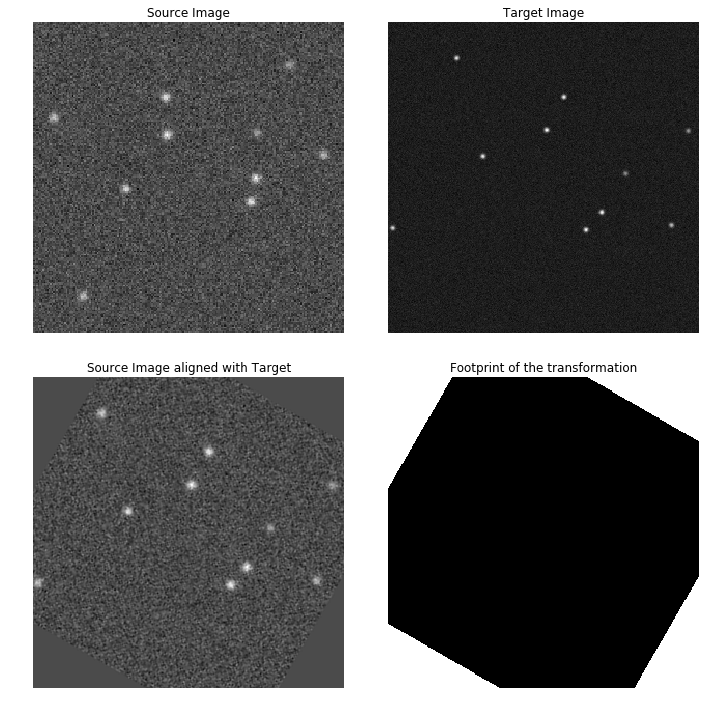# Example notebook¶

In this notebook we show how to use the main methods of astroalign.

This notebook was created for astroalign version 2.1

In :
import astroalign as aa
import numpy as np
import matplotlib.pyplot as plt
%matplotlib inline

np.random.seed(seed=12)
aa.__version__

Out:
'2.1'

## Create images¶

We will create two images of the same portion of the sky. Our source image will end up being (400, 400) pixels, have 10 stars randomly distributed. It will have a Gaussian PSF with a sigma of 2 pixels and Gaussian background noise.

The target image will be (200, 200) pixels, will be rotated 30 degrees clockwise with respect to source image. It will have a Gaussian PSF with a sigma of 1.5 pixels and Gaussian background noise.

Notice that source image is made so to have better resolution (and seeing) than the target.

In :
h, w = img_shape = (200, 200)
n_stars = 10
pos_x = np.random.randint(10, w - 10, n_stars)
pos_y = np.random.randint(10, h - 10, n_stars)
fluxes = 200.0 + np.random.rand(n_stars) * 300.0
img_posxy = np.array([[x, y] for x, y in zip(pos_x, pos_y)], dtype="float64")

img = np.zeros(img_shape)
for x, y, f in zip(pos_x, pos_y, fluxes):
img[y, x] = f

# Let's rotate and expand the original image
from scipy.ndimage import rotate, zoom
img_rotated = rotate(img, angle=30.0, reshape=False)
img_rotated = zoom(img_rotated, 1.5, order=2)

# Let's add a Gaussian PSF response with different seeing for both images
from scipy.ndimage.filters import gaussian_filter
img = gaussian_filter(img, sigma=2.0, mode='constant')
img_rotated = gaussian_filter(img_rotated, sigma=1.5, mode='constant')

# Let's add some noise to the images
noise_dc = 5.0
noise_std = np.sqrt(noise_dc)
img += np.random.normal(loc=noise_dc, scale=noise_std, size=img.shape)
img_rotated += np.random.normal(loc=noise_dc, scale=noise_std, size=img_rotated.shape)


## Solve registration with astroalign¶

In :
img_aligned, footprint = aa.register(img, img_rotated, detection_sigma=3.0)

# Try this too!! Solving using only lists of (x, y) positions
# t, __ = aa.find_transform(img_posxy, img_rotated)
# img_aligned, footprint = aa.apply_transform(t, img, img_rotated)


## Plot results¶

In :
fig, axes = plt.subplots(2, 2, figsize=(10, 10))
axes[0, 0].imshow(img, cmap='gray', interpolation='none', origin='lower')
axes[0, 0].axis('off')
axes[0, 0].set_title("Source Image")

axes[0, 1].imshow(img_rotated, cmap='gray', interpolation='none', origin='lower')
axes[0, 1].axis('off')
axes[0, 1].set_title("Target Image")

axes[1, 0].imshow(img_aligned, cmap='gray', interpolation='none', origin='lower')
axes[1, 0].axis('off')
axes[1, 0].set_title("Source Image aligned with Target")

axes[1, 1].imshow(footprint, cmap='gray', interpolation='none', origin='lower')
axes[1, 1].axis('off')
axes[1, 1].set_title("Footprint of the transformation")

axes[1, 0].axis('off')

plt.tight_layout()
plt.show()## Inquiry the parameters of the transformation before applying¶

In some occasions we need to check for the parameters of the transformation before applying it. Or maybe we just need to know the parameters for further analysis.

Another situation is where we don't have the image arrays, but we have lists of the (x, y) positions of the stars.

In these cases, we can call astroalign.find_transform.

In :
p, (pos_img, pos_img_rot) = aa.find_transform(img_posxy, img_rotated)


### Parameters of the transformation¶

In :
print("Rotation: {:.2f} degrees".format(p.rotation * 180.0 / np.pi))
print("\nScale factor: {:.2f}".format(p.scale))
print("\nTranslation: (x, y) = ({:.2f}, {:.2f})".format(*p.translation))
print("\nTranformation matrix:\n{}".format(p.params))
print("\nPoint correspondence:")
for (x1, y1), (x2, y2) in zip(pos_img, pos_img_rot):
print("({:.2f}, {:.2f}) is source --> ({:.2f}, {:.2f}) in target"
.format(x1, y1, x2, y2))

Rotation: -29.99 degrees

Scale factor: 1.50

Translation: (x, y) = (-54.67, 94.79)

Tranformation matrix:
[[  1.30097821   0.75092708 -54.66721025]
[ -0.75092708   1.30097821  94.79054671]
[  0.           0.           1.        ]]

Point correspondence:
(186.00, 114.00) is source --> (273.00, 103.32) in target
(143.00, 99.00) is source --> (205.69, 116.21) in target
(144.00, 128.00) is source --> (228.74, 153.25) in target
(59.00, 92.00) is source --> (91.24, 170.21) in target
(13.00, 138.00) is source --> (65.94, 264.55) in target
(32.00, 23.00) is source --> (4.25, 100.65) in target
(165.00, 172.00) is source --> (289.14, 194.73) in target
(86.00, 127.00) is source --> (152.53, 195.47) in target
(85.00, 151.00) is source --> (169.29, 227.37) in target
(140.00, 84.00) is source --> (190.53, 98.88) in target


### Identify corresponding stars with pos_img and pos_img_rot¶

In :
fig, axes = plt.subplots(2, 2, figsize=(10, 10))

colors = ['r', 'g', 'b', 'y', 'cyan', 'w', 'm']

axes[0, 0].imshow(img, cmap='gray', interpolation='none', origin='lower')
axes[0, 0].axis('off')
axes[0, 0].set_title("Source Image")
for (xp, yp), c in zip(pos_img[:len(colors)], colors):
circ = plt.Circle((xp, yp), 4, fill=False, edgecolor=c, linewidth=2)

axes[0, 1].imshow(img_rotated, cmap='gray', interpolation='none', origin='lower')
axes[0, 1].axis('off')
axes[0, 1].set_title("Target Image")
for (xp, yp), c in zip(pos_img_rot[:len(colors)], colors):
circ = plt.Circle((xp, yp), 4 * p.scale, fill=False, edgecolor=c, linewidth=2)